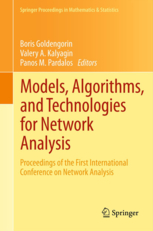• A
• A
• A
• ABC
• ABC
• ABC
• А
• А
• А
• А
• А
Regular version of the site

## An Analytical Expression for the Distribution of the Sum of Random Variables with Mixed Uniform Density and Mass Function

P. 51-63.

The distribution of the sum of independent random variables plays an important role in many problems of applied mathematics. In this paper we concentrate on the case when random variables have a continuous distribution with a discontinuity (or a probability mass) at a certain point r. Such a distribution arises naturally in actuarial mathematics when a responsibility or a retention limit is applied to every claim payment. An analytical expression for the distribution of the sum of i.i.d. random variables, which have a uniform distribution with a discontinuity, is reported.

### In bookIss. 32. NY: Springer, 2013.